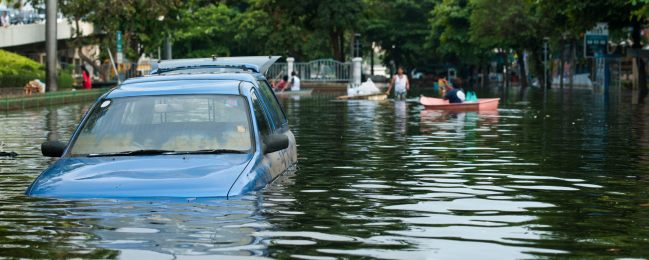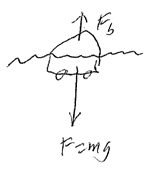## A Car Floating on Water

Advertisements for a particular brand of small car claims it would float in water if its windows were tightly closed. If the mass of the car is 1200 kg and its interior volume is 4.0m^3, what fraction of the car is submerged when it floats? Ignore the volume of the steel etc and the mass of air.Hint
$$F_{buoyancy}=(mass\: of\: water\: displaced)\times gravity$$$Hint 2 $$Density=\frac{mass}{volume}$$$
The weight of the car is counter acted by the buoyance force.
$$F_{buoyancy}=(mass\: of\: water\: displaced)\times gravity$$$$$Density=\rho_{H2O}=997\frac{kg}{m^3}=\frac{mass}{volume}$$$
Rearranging the equation to solve for mass:
$$mass=\rho \times volume$$$So, the buoyancy force expression is: $$F_{b}=volume \times \rho g=mg$$$
where $$g$$ is acceleration due to gravity, and $$m$$ is the mass. Solving for volume:
$$volume=\frac{mass}{\rho}=\frac{1200kg}{997kg/m^3}=1.2\:m^3$$$Finally, $$submerged\:fraction=\frac{1.2m^3}{4m^3}=0.3$$$
0.3